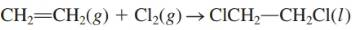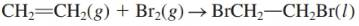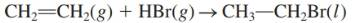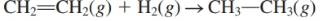Chapter 18, Problem 87AP### Introductory Chemistry: A Foundati...

9th Edition
Steven S. Zumdahl + 1 other
ISBN: 9781337399425

#### Solutions

Chapter
Section### Introductory Chemistry: A Foundati...

9th Edition
Steven S. Zumdahl + 1 other
ISBN: 9781337399425
Textbook Problem
3 views

# . Carbon compounds containing double bonds (such compounds are called alkenes) react readily with many other reagents, in each of the following reactions, identify which atoms are Oxidized and which are reduced, and specify the oxidizing and reducing agents. a.b.c.d.Interpretation Introduction

(a)

Interpretation:

The atom that oxidized, reduced, oxidizing agent and reducing agent should be determined.

Concept Introduction:

The oxidation-reduction reaction is also known as a redox reaction. In this reaction, one reactant is oxidized and other is reduced. The balancing of oxidation-reduction reaction can be done by simple method by comparing the number of each atoms on both sides of the reaction arrow without addition of hydrogen ion, water molecule and electrons from outside.

Explanation

In the given reaction, the oxidation state of chlorine on left side is 0 as in Cl2, it gets reduced to -1 in ClCH2CH2Cl. The species that oxidizes others and itself gets reduced is oxidizing agent thus, Cl2 is oxidizing agent here

Interpretation Introduction

(b)

Interpretation:

The atom that oxidized, reduced, oxidizing agent and reducing agent should be determined.

Concept Introduction:

The oxidation-reduction reaction is also known as a redox reaction. In this reaction, one reactant is oxidized and other is reduced. The balancing of oxidation-reduction reaction can be done by simple method by comparing the number of each atoms on both sides of the reaction arrow without addition of hydrogen ion, water molecule and electrons from outside.

Interpretation Introduction

(c)

Interpretation:

The atom that oxidized, reduced, oxidizing agent and reducing agent should be determined.

Concept Introduction:

The oxidation-reduction reaction is also known as a redox reaction. In this reaction, one reactant is oxidized and other is reduced. The balancing of oxidation-reduction reaction can be done by simple method by comparing the number of each atoms on both sides of the reaction arrow without addition of hydrogen ion, water molecule and electrons from outside.

Interpretation Introduction

(d)

Interpretation:

The atom that oxidized, reduced, oxidizing agent and reducing agent should be determined.

Concept Introduction:

The oxidation-reduction reaction is also known as a redox reaction. In this reaction, one reactant is oxidized and other is reduced. The balancing of oxidation-reduction reaction can be done by simple method by comparing the number of each atoms on both sides of the reaction arrow without addition of hydrogen ion, water molecule and electrons from outside.

### Still sussing out bartleby?

Check out a sample textbook solution.

See a sample solution

#### The Solution to Your Study Problems

Bartleby provides explanations to thousands of textbook problems written by our experts, many with advanced degrees!

Get Started